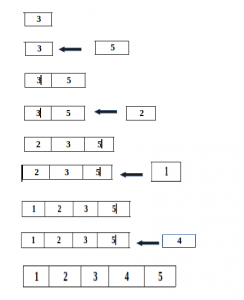# Online Algorithm

An online algorithm is one that can process its input piece-by-piece in a serial fashion, i.e., in the order that the input is fed to the algorithm, without having the entire input available from the beginning.

In contrast, an offline algorithm is given the whole problem data from the beginning and is required to output an answer which solves the problem at hand.

As an example, consider the sorting algorithms selection sort and insertion sort:

The selection sort algorithm sorts an array by repeatedly finding the minimum element (considering ascending order) from unsorted part and putting it at the beginning. which requires access to the entire input; it is thus an offline algorithm. On the other hand, insertion sort considers one input element per iteration and produces a partial solution without considering future elements. Thus insertion sort is an online algorithm.

EXAMPLE OF ONLINE ALGORITHM (INSERTION SORT):Because an online algorithm does not know the whole input, it might make decisions that later turn out not to be optimal,
Note that insertion sort produces the optimum result. Therefore, for many problems, online algorithms cannot match the performance of offline algorithms.

Example of Online Algorithms are :
1. Insertion sort
2. Perceptron
3. Reservoir sampling
4. Greedy algorithm
7. Odds algorithm

Online Problems: There are many problems that offer more than one online algorithm as solution:
2. Linear Search Problem
3. K-server problem
4. Job shop scheduling problem
5. List update problem
6. Bandit problem
7. Secretary problem

This article is contributed by Shubham Rana. If you like GeeksforGeeks and would like to contribute, you can also write an article using contribute.geeksforgeeks.org or mail your article to contribute@geeksforgeeks.org. See your article appearing on the GeeksforGeeks main page and help other Geeks.